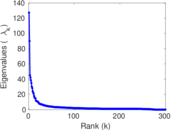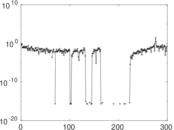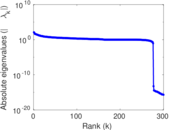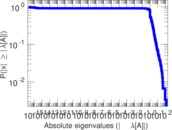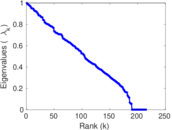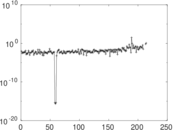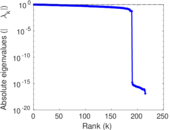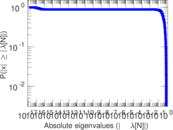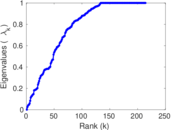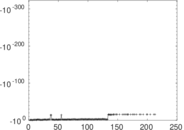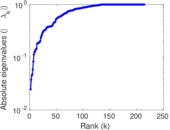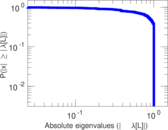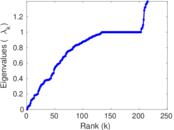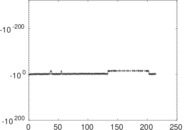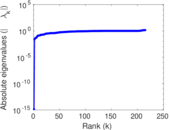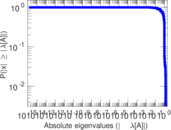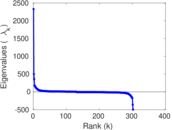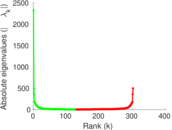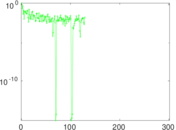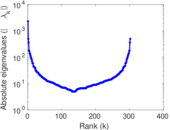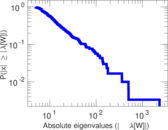# Wikibooks edits (tl)

This is the bipartite edit network of the Tagalog Wikibooks. It contains users and pages from the Tagalog Wikibooks, connected by edit events. Each edge represents an edit. The dataset includes the timestamp of each edit.

 Code `btl` Internal name `edit-tlwikibooks` Name Wikibooks edits (tl) Data source http://dumps.wikimedia.org/ AvailabilityDataset is available for download Consistency checkDataset passed all tests Category Authorship network Dataset timestamp 2017-10-20 Node meaning User, article Edge meaning Edit Network formatBipartite, undirected Edge typeUnweighted, multiple edges Temporal dataEdges are annotated with timestamps

## Statistics

 Size n = 2,341 Left size n1 = 302 Right size n2 = 2,039 Volume m = 5,767 Unique edge count m̿ = 3,224 Wedge count s = 320,325 Claw count z = 45,869,085 Cross count x = 6,262,846,249 Square count q = 27,487 4-Tour count T4 = 1,509,644 Maximum degree dmax = 1,125 Maximum left degree d1max = 1,125 Maximum right degree d2max = 184 Average degree d = 4.926 95 Average left degree d1 = 19.096 0 Average right degree d2 = 2.828 35 Fill p = 0.005 235 65 Average edge multiplicity m̃ = 1.788 77 Size of LCC N = 1,951 Diameter δ = 14 50-Percentile effective diameter δ0.5 = 3.740 12 90-Percentile effective diameter δ0.9 = 5.918 83 Median distance δM = 4 Mean distance δm = 4.467 41 Gini coefficient G = 0.737 002 Balanced inequality ratio P = 0.208 167 Left balanced inequality ratio P1 = 0.119 993 Right balanced inequality ratio P2 = 0.293 567 Relative edge distribution entropy Her = 0.809 101 Power law exponent γ = 3.432 90 Tail power law exponent γt = 2.511 00 Tail power law exponent with p γ3 = 2.511 00 p-value p = 0.000 00 Left tail power law exponent with p γ3,1 = 1.751 00 Left p-value p1 = 0.753 000 Right tail power law exponent with p γ3,2 = 2.961 00 Right p-value p2 = 0.061 000 0 Degree assortativity ρ = −0.268 462 Degree assortativity p-value pρ = 2.413 65 × 10−54 Spectral norm α = 127.356 Algebraic connectivity a = 0.024 568 8 Spectral separation |λ1[A] / λ2[A]| = 1.414 24 Controllability C = 1,628 Relative controllability Cr = 0.746 789

## Plots

### Fruchterman–Reingold graph drawing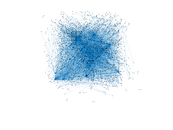### Degree distribution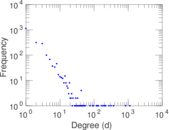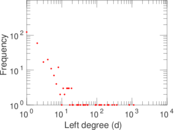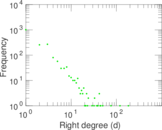### Cumulative degree distribution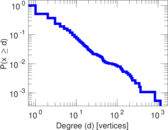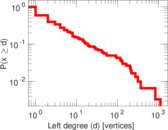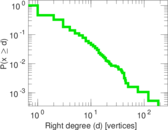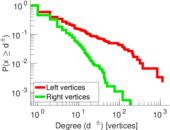### Lorenz curve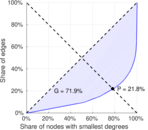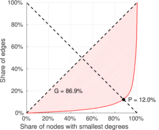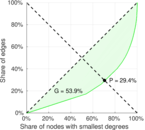### Spectral distribution of the adjacency matrix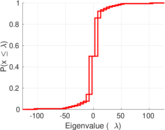### Spectral distribution of the normalized adjacency matrix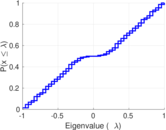### Spectral distribution of the Laplacian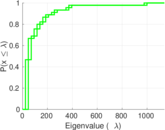### Spectral graph drawing based on the adjacency matrix### Spectral graph drawing based on the Laplacian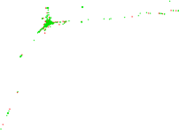### Spectral graph drawing based on the normalized adjacency matrix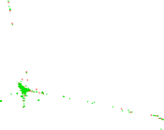### Degree assortativity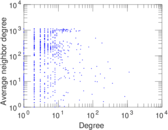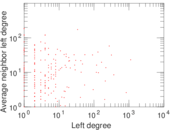### Zipf plot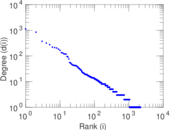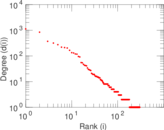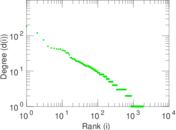### Hop distribution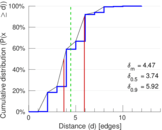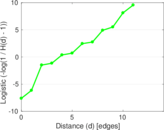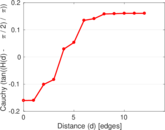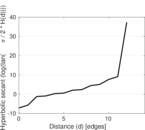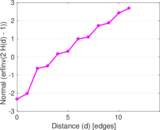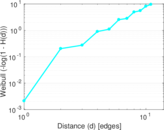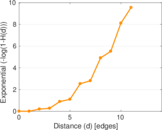### Double Laplacian graph drawing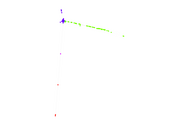### Delaunay graph drawing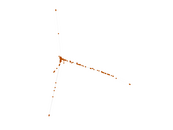### Edge weight/multiplicity distribution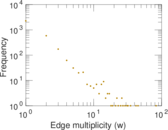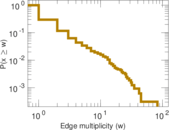### Temporal distribution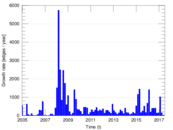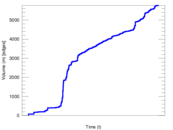### Temporal hop distribution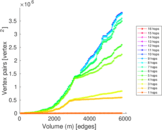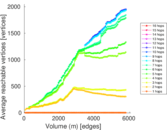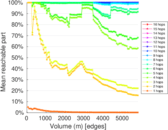### Diameter/density evolution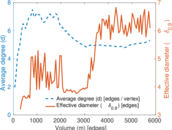### Matrix decompositions plots